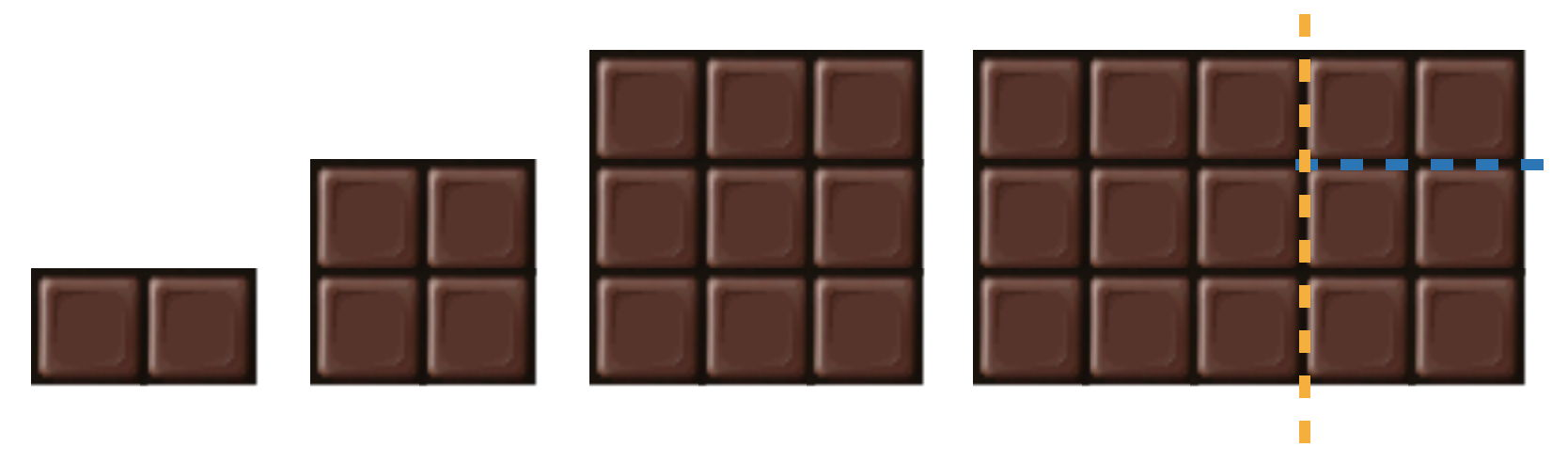시간 제한메모리 제한제출정답맞힌 사람정답 비율
2 초 (추가 시간 없음) 1024 MB111100.000%

## 문제

Selma is visited by her two grandchildren Elsa and Asle who love chocolate. To be precise, they are especially fond of the brand Nut Cream Puffed Chocolate that comes in bars made up by $6 \times 6$ squares. The bars can be broken along the valleys between squares into smaller rectangular bars of integer dimensions. Due to the fragile nature of this type of chocolate, the bars often break into smaller rectangular bars even before you unpack them (but still only of integer dimensions).

Thus Selma finds herself with a set of rectangular bars of various dimensions in her candy stash. She knows how important it is to be fair to children, so not only does she want to give Elsa and Asle the same amount of chocolate, but also identical collections of rectangular bars (where an $a \times b$ bar is considered identical to a $b \times a$ bar).  To do this, Selma can break her bars into smaller pieces.  A break is the operation of taking an $a\times b$ bar and breaking it along a valley to produce two bars of dimensions $c\times b$ and $(a-c)\times b$, for some integer $c\in [1,a-1]$, or two bars of dimensions $a\times d$ and $a\times (b-d)$, for some integer $d\in [1,b-1]$.  See Figure B.1 for an example.

Selma would like to give her two grandchildren identical collections of bars, each collection consisting of at least $t$ squares of chocolate.  What is the minimum number of breaks she needs to make to be able to do this?Figure B.1: Explanation of Sample Input 1. First make a vertical break as shown on the $3 \times 5$ bar (orange), then make a horizontal break on the newly created $3 \times 2$ bar (blue). This way Elsa and Asle can each get one $1 \times 2$, one $2 \times 2$, and one $3 \times 3$ bar, in total $15$ squares each.

## 입력

The first line of input contains two integers $n$ and $t$ ($1 \le n \le 50$, $1 \le t \le 900$), where $n$ is the number of bars Selma has, and $t$ is the least number of squares she wants each grandchild to receive. Then follows a line containing $n$ bar descriptions. A bar description is on the format "$a$x$b$" for two integers $1 \le a, b \le 6$.

You may assume that the total amount of chocolate squares among the $n$ bars is at least $2t$.

## 출력

Output the minimum number of breaks needed to obtain two identical collections of bars, each having a total of at least $t$ squares.

## 예제 입력 1

4 15
1x2 2x2 3x3 3x5


## 예제 출력 1

2


## 예제 입력 2

6 7
1x2 2x3 1x4 3x2 4x1 6x6


## 예제 출력 2

0


## 예제 입력 3

5 3
1x1 1x1 1x1 1x1 1x4


## 예제 출력 3

1


## 출처• 문제를 만든 사람: Andreas Björklund, Mats Petter Wallander